Home > A2C > Chapter 7 > Lesson 7.3.2 > Problem7-177

7-177.
1. Solve each of the following equations. Homework Help ✎

1. (x + 4)(2x − 5) = 0

2. (x + 4)(x2 − 5x + 6) = 0

3. 3x(x + 1)(2x − 7)(3x + 4)2(x − 13)(x + 7) = 0

4. Describe how to solve an equation made up of any number of factors all multiplied to equal zero.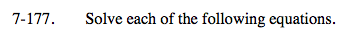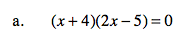Use the Zero Product Property.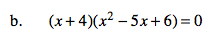Factor and use the Zero Product Property.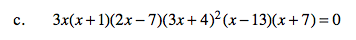See parts (a) and (b).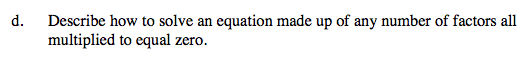What did you do to solve the equations in parts (a)-(c)?